# Motion in plane Problems with Solutions for JEE Main & JEE Advanced

In this page we have 2-D Kinematics/Motion in plane Problems with Solutions for JEE Main & JEE Advanced .This MCQ exercise has questions about vectors, projectile and two dimensional motion. Hope you like them and do not forget to like , social share and comment at the end of the page.

## JEE Muliple Choice Questions

Question 1.
The radius vector of point x relative to origin varies with time as
$\mathbf{r}=a cos(kt)\mathbf{i}+b sin(kt)\mathbf{j}$
Where a and b are constants and i and j are vectors along x and y axis. Which one of the following is the mean velocity vector?
a. $\frac {a cos(kt)\mathbf{i}- b sin(kt)\mathbf{j}}{t}$
b. $a cos(kt)\mathbf{i}+ b sin(kt)\mathbf{j}$
c. $a cos(kt)\mathbf{i}- b sin(kt)\mathbf{j}$
d. $\frac {a cos(kt)\mathbf{i}+ b sin(kt)\mathbf{j}}{t}$

Question 2.
A wind is blowing in the North direction at the speed of 5 km/hr. An airplane moves to a point in the East which is 2000 km away in 40 hr. Find the velocity of the airplane with respect to the wind.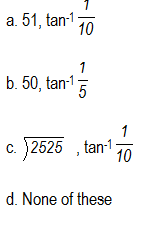Question 3.
Two cars A and B run at constant speed $u_1$ and $u_2$ along the highways intersecting at an angle $\theta$. They start at t=0 at the intersection point. Find the time required to have distance s between the two cars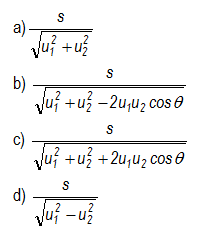Question 4.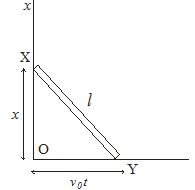A bar XY of length l which always remains in the same vertical plane has its ends X and Y constrained to remain in contact with the horizontal floor and in vertical wall as shown below in the figure.
The bar starts from a vertical position and end X is moved along the floor with a constant velocity v0 so that its displacement OX=v0t. The displacement time graph for Y end is
(a) Ellipse
(b) Circle
(c) Parabola
(d) Straight line

Question 5.
Three particles of mass m1,m2,m3 moves in plane with initial velocity v1 , v2 , v3 . A constant acceleration a acts on the three particles. At t=t0, there velocities becomes v1', v2' , v3' Which of the following expression are correct?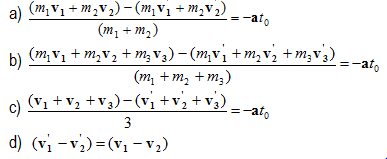Question 6.
Two particles X and Y travel along the x and y axis with respective velocities
$\mathbf{v_1} = 2\mathbf{i}$ m/sec
$\mathbf{v_2} = 3\mathbf{j}$ m/sec
At t=0 they are at
$x_1 = -3m$ and $y_1=0$
$x_2= 0$ and $y_2=-3 m$
Find the vector which represents the position of Y relative to X as a function of t
i and j are respective unit vectors along x and y direction
a. $(3t -3) \mathbf{j} + (3-2t) \mathbf{i}$
b. $(3t +3) \mathbf{j} + (3+2t) \mathbf{i}$
c. $(3t -2) \mathbf{j} + (2-2t) \mathbf{i}$
d. None of the above

(B) Two ring X and Y are put on two vertical stationary rods AB and AB. An inextensible thread is fixed at point A and on ring X and is passed through ring Y.
The system is shown below in Figure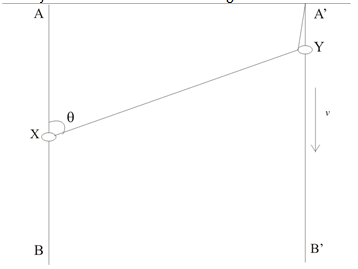Question 7
The ring X moves with constant velocity v downwards. The angle AXY is θ. Find the velocity of the ring Y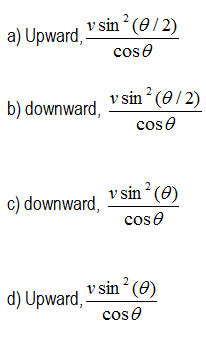Question 8
Find the velocity of ring Y with respect to ring X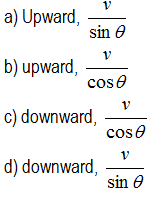(C)
A man X can swim at a speed of 10 m/s with respect to river . He wants to cross the river which is 50 m wide and has a current of 5 m/s
If he wishes to land on the other bank at a point directly across the river from his starting point

Question 9
Velocity of man with respect to the person standing on the bank
a. 5 m/s
b. 10 m/s
c. 6 m/s
d. $5 \sqrt {3}$ m/s

Question 10
Find the angle made by the man from the current
a. 120°
b. 60°
c. 30°
d. 90°

Question 11
Find the time taken by the man to cross the river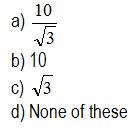(D)Now if he instead decides to cross the river in shortest possible time
Question 12
what direction it should swim
a. 90° to current
b. 30° to current
c. 60 ° to current
d. none of these

Question 13.
How much time
a. 5
b. 10
c. 6
d. 4

Question 14.
How much distance it will land from his starting point
a. 30
b. 20
c. 25
d. none of these

(E)The radius vector describing the position of the particle A relative to origin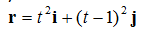Question 15.
Find the rectangular components of the average velocity in the time interval between $t$ and $t + \Delta t$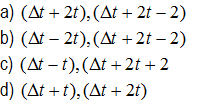Question 16.
Which of the following statements are true about the motion
a. The particle is experiencing uniform acceleration motion
b. The particle starts at y axis and touched x axis in 1sec
c. The initial velocity is towards negative Y axis
d. The velocity at t=1 is towards positive x axis

(F)
A particle is moving along a parabola given by
$y=x^2$
So that at any time $v_x=3 m/s$

Question 17.
Find the magnitude and direction of velocity at x=1/3 m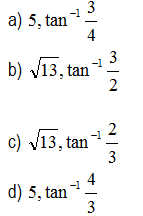Question 18
Find the acceleration at x=1/3 (both magnitude and direction)
a. 18 m/sec2 ,90°
b. 14 m/sec2 ,60°
c. 18 m/sec2 ,60°
d. 14 m/sec2 ,90°